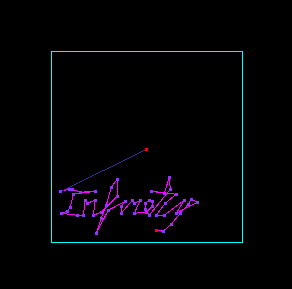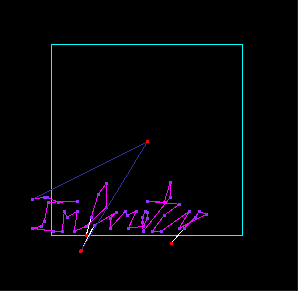# 14th of April 2022- infinitely stupid…

I am pretty sure I overthink things and/or I am to stupid for this.

I have abandoned the notion of a fixed scale. Having a fixed scale and having to double/tripple/quadrupel vectors or moves and discerning whether I can add (or substract) something or not… was really (REALLY) messy.

So I started new with “fixed” strengths and an adjustable scale.
Just updating the scale is on one hand easier – since I do not have to discern between adding/subtracting from the position in regard to the quadrant – the list gets larger in the right direction automatically.

Reminder – what are our restrictions:

• 8 bit machine, a “strength” can only be between -128 and +127
• RAM, we only have about 800 byte of RAM
• our vectors reside in some sort of list and for performance reason should be drawn one after another (-> most lines are “delta” lines, not “absolut” lines) one entity of vectors that are drawn after one another I call scene or scenario
• largest “sensible” scale is 0xff

Other challenges still persist:

• it is possible for a scenario when expanding to get out of bounds “within” the scenario -> there is a need to split a scenario
• a split scenario has to be properly positioned after the split (meaning there is a Reset0Ref and a positioning)
• for that to be possible one has to keep track of a global “absolut” position and transform these into a move from zero
• a split scenario can result in a scenario of only “move” vectors -> which than must be deleted
• a scale can get higher than 0xff – how to handle that?
if we split the vector we have again the fact that with each split the zoom doubles
if we double the strength and half the scale and than continue to scale -> it also doubles the zoom

-> Scenarios must be build such that following is ensured:

• that no move/line will every need to have a scale higher 0xff

One major problem is, that all positioning/size on screen display on a vectrex is build of two components:

• scale
• strength

As long as both are variable it is nearly impossible to do something exact. But keeping one constant for all that is needed is equally impossible – imagine this:Infinity inside the 8bit boundary – placed with a move vector

There is only one move-vector – with a relatively large scale and line-vectors with a relative small scale.
Data wise this looks like:

```Reset Move
Move ( \$C9CD ): \$B0 , \$C0 - scale: \$D006=\$AA
Line ( \$C88F ): \$02 , \$12 - scale: \$D006=\$4B
Line ( \$C895 ): \$00 , \$06 - scale: \$D006=\$4B
Line ( \$C89B ): \$FA , \$10 - scale: \$D006=\$4B
Line ( \$C8A1 ): \$02 , \$1F - scale: \$D006=\$4B
Line ( \$C8A7 ): \$FC , \$D3 - scale: \$D006=\$4B
Line ( \$C8AD ): \$E4 , \$FA - scale: \$D006=\$4B
Line ( \$C8B3 ): \$F9 , \$FA - scale: \$D006=\$4B
Line ( \$C8B9 ): \$FC , \$F4 - scale: \$D006=\$4B
Line ( \$C8BF ): \$FC , \$1F - scale: \$D006=\$4B
Line ( \$C8C5 ): \$01 , \$0E - scale: \$D006=\$4B
Line ( \$C8CB ): \$1C , \$03 - scale: \$D006=\$4B
Line ( \$C8D1 ): \$FA , \$04 - scale: \$D006=\$4B
Line ( \$C8D7 ): \$06 , \$10 - scale: \$D006=\$4B
Line ( \$C8DD ): \$E4 , \$FC - scale: \$D006=\$4B
Line ( \$C8E3 ): \$06 , \$10 - scale: \$D006=\$4B
Line ( \$C8E9 ): \$1F , \$20 - scale: \$D006=\$4B
Line ( \$C8EF ): \$22 , \$00 - scale: \$D006=\$4B
Line ( \$C8F5 ): \$F0 , \$F4 - scale: \$D006=\$4B
Line ( \$C8FB ): \$DC , \$F5 - scale: \$D006=\$4B
Line ( \$C901 ): \$E6 , \$F8 - scale: \$D006=\$4B
Line ( \$C907 ): \$E2 , \$F5 - scale: \$D006=\$4B
---F---
Line ( \$C90D ): \$2E , \$18 - scale: \$D006=\$4B
Line ( \$C913 ): \$12 , \$24 - scale: \$D006=\$4B
Line ( \$C919 ): \$F6 , \$F6 - scale: \$D006=\$4B
Line ( \$C91F ): \$F2 , \$01 - scale: \$D006=\$4B
...```

That data is essentially the “round” before the “F” split.

After zooming a bit … it looks essentially:broken – due to removed out of bounds vectors – but onscreen “ok”

Following data is exactly after the first split… the “F” split:

```Reset Move
Move ( \$C9CD ): \$B0 , \$C0 - scale: \$D006=\$AB
Line ( \$C88F ): \$02 , \$12 - scale: \$D006=\$4C
Line ( \$C895 ): \$00 , \$06 - scale: \$D006=\$4C
Line ( \$C89B ): \$FA , \$10 - scale: \$D006=\$4C
Line ( \$C8A1 ): \$02 , \$1F - scale: \$D006=\$4C
Line ( \$C8A7 ): \$FC , \$D3 - scale: \$D006=\$4C
Line ( \$C8AD ): \$E4 , \$FA - scale: \$D006=\$4C
Line ( \$C8B3 ): \$F9 , \$FA - scale: \$D006=\$4C
Line ( \$C8B9 ): \$FC , \$F4 - scale: \$D006=\$4C
Line ( \$C8BF ): \$FC , \$1F - scale: \$D006=\$4C
Line ( \$C8C5 ): \$01 , \$0E - scale: \$D006=\$4C
Line ( \$C8CB ): \$1C , \$03 - scale: \$D006=\$4C
Line ( \$C8D1 ): \$FA , \$04 - scale: \$D006=\$4C
Line ( \$C8D7 ): \$06 , \$10 - scale: \$D006=\$4C
Line ( \$C8DD ): \$E4 , \$FC - scale: \$D006=\$4C
Line ( \$C8E3 ): \$06 , \$10 - scale: \$D006=\$4C
Line ( \$C8E9 ): \$1F , \$20 - scale: \$D006=\$4C
Line ( \$C8EF ): \$22 , \$00 - scale: \$D006=\$4C
Line ( \$C8F5 ): \$F0 , \$F4 - scale: \$D006=\$4C
Line ( \$C8FB ): \$DC , \$F5 - scale: \$D006=\$4C
Line ( \$C901 ): \$E6 , \$F8 - scale: \$D006=\$4C
Line ( \$C907 ): \$E2 , \$F5 - scale: \$D006=\$4C
---F---
Reset Move
Move ( \$C9D3 ): \$A9 , \$E9 - scale: \$D006=\$E6
Line ( \$C90D ): \$2E , \$18 - scale: \$D006=\$4C
Line ( \$C913 ): \$12 , \$24 - scale: \$D006=\$4C
Line ( \$C919 ): \$F6 , \$F6 - scale: \$D006=\$4C
Line ( \$C91F ): \$F2 , \$01 - scale: \$D006=\$4C```

After some more steps the display (without further cropping) will look like this:

The “inety” will zoom “slower” now that the “Inf”.
The reason for that is… the “old” F-Zoom was a mixture of the original move (scale \$AA) and the other vectors (scale \$4B).
The new F-Zoom is a mixture of the new move (scale \$E6) and the other vectors (scale \$4C).
(scale values updated every round).

The thing is as soon as I calculate a new new move for a new split – the future factorization will be different and the scenes will drift apart”.

But I HAVE to calculate the new move… otherwise I would have to reuse the old scale… and doing that for langer than \$ff cycles will:
– a take really long!
– the scale will sooner or later be larger than \$ff – and where would it end? – Never! I MUST reduce the scale at some point!

I will try to work with these features and see where it gets me – but it will never be totally “clean”.
I should do Infinity Dive on a PiTrex – that would look like the real thing – on the vectrex itself it won’t.

… anyway I’ll see where I get with this.

This site uses Akismet to reduce spam. Learn how your comment data is processed.